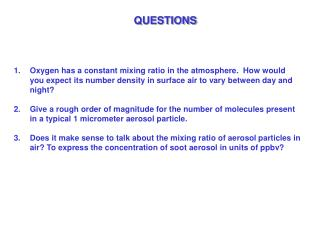# QUESTIONS - PowerPoint PPT PresentationDownload PresentationQUESTIONS

QUESTIONSDownload Presentation## QUESTIONS

- - - - - - - - - - - - - - - - - - - - - - - - - - - E N D - - - - - - - - - - - - - - - - - - - - - - - - - - -
##### Presentation Transcript

1. QUESTIONS • Oxygen has a constant mixing ratio in the atmosphere. How would you expect its number density in surface air to vary between day and night? • Give a rough order of magnitude for the number of molecules present in a typical 1 micrometer aerosol particle.  • Does it make sense to talk about the mixing ratio of aerosolparticles in air? To express the concentration of soot aerosol in units of ppbv?

2. 2. PHYSICAL CHEMISTRY BASICS / KINETICS(CHAPTER 9 +)

3. THE PERIODIC TABLE First drafts of Mendeleev’s periodic table, 1869 photos from Mendeleev museum, St. Petersburg, 2007

4. CHEMICAL BONDS Bond formation involves the electrons (e-) in the outermost (valence) shell. A complete outer shell consists of 8 valence electrons (except H and He which have 2) Destruction of a bond corresponds to a release of energy. Generally double or triple bond energies are higher than for single bonds. Ionic bonds: electron attraction between positive and negative ions  e- transfer Convalent bonds: sharing of paired electrons Polar convalent bonds: When 2 atoms from different elements share e- unequally

5. OXIDATION STATE Oxidation State describes positive or negative character of atoms, or degree of oxidation Ionic Molecules: oxidation state is the same as the charge on the ion example: Na+1Cl-1 Ca+2Br2-1 Note: sum of oxidation numbers must equal zero Covalent Molecules: more arbitrary, based on electronegativity scale example: CO2: C+4O2-2  oxidation: C oxidation state has increased from -IV to +IV (the opposite would be reduction) Atmosphere is generally an oxidizing medium.

6. ORGANIC MOLECULAR NOMENCLATURE Alkanes (C-C single bonds) Alkenes (C-C double bonds) CnH2n+2 CnH2n ethane ethene Alkynes (C-C triple bonds) Aromatic compounds CnH2n-2 CnH2n-6 ethyne Benzene Oxygenated hydrocarbons: Aldehydes, alcohols, ketones, etc… acetaldehyde methanol Acetic acid

7. COMMON IONS Ammonium NH4+ Acetate CH3COO- Nitrate NO3- Nitrite NO2- Hydroxide OH- Hypochlorite ClO- Chlorite ClO2- Chlorate ClO3- Perchlorate ClO4- Permanganate MnO4- Carbonate CO32- Sulfate SO42- Sulfite SO32- Peroxide O22- Silicate SiO32- Phosphate PO43-

8. CHEMICAL THERMODYNAMICS Enthalpy: Thermodynamic potential of the system Heat of reaction (ΔHrxn)= change of enthalpy  depends on T, is independent of path ΔHf = heat of formation (per mole) by definition = 0 for elements Exothermic Endothermic Gibbs Free Energy:  calculated ΔG in same way as enthalpy change ΔG < 0  forward reaction spontaneous ΔG > 0  reverse reaction spontaneous ΔG = 0  reaction is at equilibrium S = entropy

9. REACTION RATES: BASICS A balanced chemical reaction does not represent the actual steps of thereaction pathway or mechanism Rate-determining step: the slowest step which determines the max rate of overall rxn Rate of an elementary reaction: A + B  C Reaction Rate = k [A][B] Rate of reactions generally increase with temperature: Catalysts decrease the energy of activation  increases the rate of forward and reverse reactions k=rate constant If A ≠ f(T) = Arrhenius form E = activation energy a,b correspond to reaction order General Reaction Rates: aA + bB + …  gG + hH …. , k Reaction Rate = k[A]a[B]b…

10. CHEMICAL KINETICS For multi-step reactions, need to sum the individual reaction rates: A + B  C k1 A + D  B k2 Biomolecular Reaction: A + B  C + D Collision of 2 reactants (A and B) forms an activated complex (AB*) which decomposes rapidly to the products (C and D) Reaction Rate: Special Case: Self Reaction: A + A  B + C k: unit here [cm3/molecule/s]

11. CHEMICAL KINETICS: THREE-BODY REACTIONS A + B  AB* 3 AB*  A + B 4 AB* + M  AB + M* 5 M*  M + heat 6 A + B + M  AB + M 7 M = third body (usually inert: O2, N2)  stabilizes the excited products AB* In the atmosphere, take [M]=na Rate of formation from 3rd rxn: But assume AB* short lifetime, can use steady state approximation formation rate = loss rate Re-arrange: Low-pressure limit [M] << k4/k5: rate depends linearly on [M] High-pressure limit [M] >> k4/k5 rate independent of [M] (all AB* will stabilize) R3 is the rate-limiting step

12. CHEMICAL EQUILIBRIA Notation: also see kr=k-f A + B  C + D, kf A + B ↔ C + D C + D  A + B, kr At equilibria (or ss) : Reaction Quotient (not in equilibrium): if Q < Keq then rxn will shift to R (more products) if Q > Keq then rxn will shift to L (more reactants) Le Châtelier’s Principle: Perturbance of a system at equilibrium  system will shift to minimize perturbance

13. PHOTOLYSIS Breaking a chemical bond with an incident photon: AB + hν A + B AB + hν AB* AB + hνluminescence  AB + M quenching  A + B photodissociation j = photolytic rate constant [s-1] h = Planck constant ν = frequency J = actinic flux [photons/cm2/s] σx = absorption cross-section [cm2/molecule] φx = quantum yield (probability photon abs causes photolysis) [molecules/photon] Defining the photolytic rate constant: For polycromatic radiation: A gas molecule will absorb radiation at a given wavelength only if the energy can be used to increase the internal energy of a molecule Rotational transitions  far IR radiation (> 20 µm) Vibrational transitions  near IR radiation (0.7-20 µm) Electronic transitions  UV radiation (< 0.4 µm)